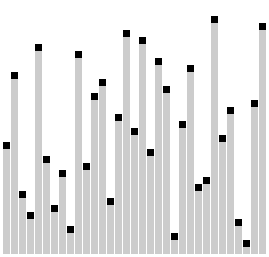# PHP梳排序（Comb Sort）算法的实现PHP梳排序示意图如下：```<?php
function combSort(\$my_array){
\$gap = count(\$my_array);
\$swap = true;
while (\$gap > 1 || \$swap){
if(\$gap > 1) \$gap /= 1.25;
\$swap = false;
\$i = 0;
while(\$i+\$gap < count(\$my_array)){
if(\$my_array[\$i] > \$my_array[\$i+\$gap]){
list(\$my_array[\$i], \$my_array[\$i+\$gap]) = array(\$my_array[\$i+\$gap],\$my_array[\$i]);
\$swap = true;
}
\$i++;
}
}
return \$my_array;
}
\$test_array = array(3, 0, 2, 5, -1, 4, 1);
echo "原始数组 :\n";
echo implode(', ',\$test_array );
echo "\n排序后数组\n:";
echo implode(', ',combSort(\$test_array)). PHP_EOL;```

```原始数组 : 3, 0, 2, 5, -1, 4, 1